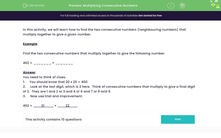# Multiplying Consecutive Numbers

In this worksheet, students find two consecutive numbers which multiply to a given number. They round numbers so that they can calculate their answers mentally.Key stage:  KS 3

Curriculum topic:   Number

Curriculum subtopic:   Use Approximation to Estimate/Calculate Answers

Difficulty level:#### Worksheet Overview

In this activity, we will learn how to find the two consecutive numbers (neighbouring numbers) that multiply together to give a given number.

Example

Find the two consecutive numbers that multiply together to give the following number.

462 = _______ × _______

You need to think of clues.

1.     You should know that 20 x 20 = 400

2.     Look at the last digit, which is 2 here.  Think of consecutive numbers that multiply to give a final digit of 2.  They are 1 and 2 or 3 and 4 or 6 and 7 or 8 and 9.

3.     Now use trial and improvement.

462 =          21           ×          22

### What is EdPlace?

We're your National Curriculum aligned online education content provider helping each child succeed in English, maths and science from year 1 to GCSE. With an EdPlace account you’ll be able to track and measure progress, helping each child achieve their best. We build confidence and attainment by personalising each child’s learning at a level that suits them.

Get started